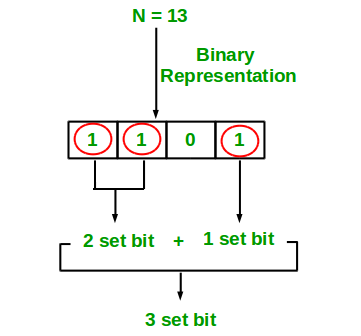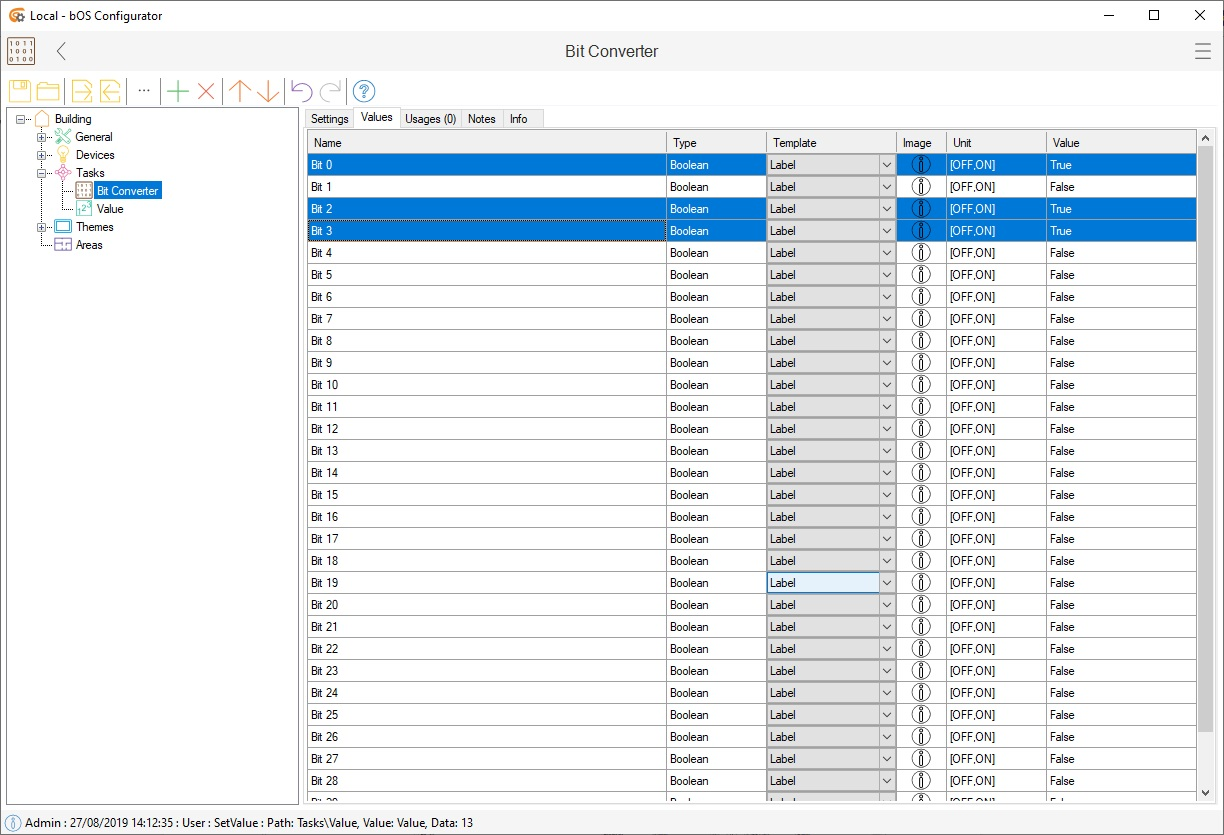# Bit Converter

Converts integer values to bits, which can be used for another home automation device.

## Bit converter

Bit converter converts integer input values to bits, which can be used for another home automation device. Conversion from integer into bit value is done by the standard bit conversion protocol (using binary bits 0 and 1). Additional information on the conversion is shown in the picture 1, where value 13 is converted into bits.Picture 1: Bit conversion explanation

Each of these bits can be used to add additional functionalities into the building. Picture 2 shows how integer value 13 is displayed using bit converter in bOS.Picture 2: Bit converter